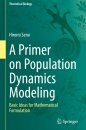United States
£ GBP
EU Shipping Update - read more

## British Wildlife

8 issues per year 84 pages per issue Subscription only

British Wildlife is the leading natural history magazine in the UK, providing essential reading for both enthusiast and professional naturalists and wildlife conservationists. Published eight times a year, British Wildlife bridges the gap between popular writing and scientific literature through a combination of long-form articles, regular columns and reports, book reviews and letters.

Subscriptions from £32 per year

## Conservation Land Management

4 issues per year 44 pages per issue Subscription only

Conservation Land Management (CLM) is a quarterly magazine that is widely regarded as essential reading for all who are involved in land management for nature conservation, across the British Isles. CLM includes long-form articles, events listings, publication reviews, new product information and updates, reports of conferences and letters.

Subscriptions from £22 per year

# A Primer on Population Dynamics Modeling Basic Ideas for Mathematical Formulation

Textbook
By: Hiromi Seno(Author)
462 pages, b/w illustrations
Publisher: Springer Nature
Click to have a closer look
•ISBN: 9789811960154 Hardback Nov 2022 Not in stock: Usually dispatched within 1-2 weeks
£89.99
#261657

This textbook provides an introduction to the mathematical models of population dynamics in mathematical biology. The focus of this book is on the biological meaning/translation of mathematical structures in mathematical models, rather than simply explaining mathematical details and literacies to analyze a model. In some recent usages of the mathematical model simply with computer numerical calculations, the model includes some inappropriate mathematical structure concerning the reasonability of modeling for the biological problem under investigation. For students and researchers who study or use mathematical models, it is important and helpful to understand what mathematical setup could be regarded as reasonable for the model with respect to the relation between the biological factors involved in the assumptions and the mathematical structure of the model.

Topics covered in this book are; modeling with geometric progression, density effect in population dynamics, deriving continuous time models from discrete time models, basic modeling for birth-death stochastic processes, continuous time models, modeling interspecific reaction for the continuous time population dynamics model, competition and prey-predator dynamics, modeling for population dynamics with a heterogeneous structure of population, qualitative analysis on the discrete time dynamical system, necessary knowledge about fundamental mathematical theories to understand the dynamical nature of continuous time models. The book includes popular topics in ecology and mathematical biology, as well as classic theoretical topics.

By understanding the biological meaning of modeling for simple models, readers will be able to derive a specific mathematical model for a biological problem by reasonable modeling. The contents of this book is made accessible for readers without strong mathematical background.

## Contents

Part I. Modeling Biological Factors
Chapter 1. Application of Geometric Progression
Chapter 2. Influence From Surrounding
Chapter 3 .From Discrete Time Model to Continuous Time Model
Chapter 4 Continuous Time Modeling for Birth and Death Processes
Chapter 5. Continuous Time Modeling for Single Species Population Dynamics
Chapter 6. Modeling of Interspecific Reaction
Chapter 7. Modeling for Competitive Relation
Chapter 8. Modeling for Prey-Predator Relation
Chapter 9. Modeling with Class Structure
Chapter 10. Modeling for Age Structure

Part II. Mathematical Equipments
Chapter 11. Homogeneous Linear Difference Equation
Chapter 12. Qualitative Analysis for Discrete Time Model
Chapter 13. First Order Linear Ordinary Differential Equation
Chapter 14. Qualitative Analysis for Continuous Time Model
Chapter 15. Essentials of Poisson Process/Distribution

## Customer Reviews

Textbook
By: Hiromi Seno(Author)
462 pages, b/w illustrations
Publisher: Springer Nature